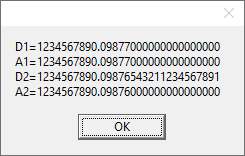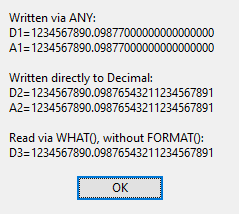# Caveat when using ANY &= DECIMAL

Was testing some code in one of my classes, and discovered something: When you assign a DECIMAL containing a lot of places to an ANY, you lose precision when accessing it via read or write.
When you write to it, it apparently rounds off. When you read the data, it truncates.``````PROGRAM

MAP
END

A1  ANY
A2  ANY
D1  DECIMAL(30,20)
D2  DECIMAL(30,20)

CODE

A1 &= D1
A2 &= D2
A1  = 1234567890.09876543211234567891
D2  = 1234567890.09876543211234567891
MESSAGE('D1=' & FORMAT(D1,@N31.20) & '|A1=' & FORMAT(A1,@N31.20) & '|D2=' & FORMAT(D2,@N31.20) & '|A2=' & FORMAT(A2,@N31.20))``````

Looks like that’s what’s happening.

I tried a REAL and it had the expected precision. I wonder if is some kind of base type thing which ANY base type is REAL or LONG but never Decimal.

I also tried an &DECIMAL and see the same problem that only 6 decimals of 10 come out (DRef2 below &= D2). That seems even more surprising and a Bug. Maybe I’m missing something? I revised the test to take A2 out so D2 had no interaction with the ANY. I’ll try this in C10 tomorrow.

One ANY thing that catches me is in `CHOOSE(#,Any,'String')` an ANY is treated as a NUMBER when =‘String’ so CHOOSE() has mixed types and returns 0. I make ANY a String with clip() as `CHOOSE(#,clip(Any),'String')`

``````AnyDecimalJS PROCEDURE()
A1  ANY
A2  ANY
D1  DECIMAL(30,20)
D2  DECIMAL(30,20)
Real1 REAL
AnyR1 ANY
DRef2 &DECIMAL
D3     DECIMAL(30,20)
DRef3 &DECIMAL
CODE

A1 &= D1
A2 &= D2
A1  = 1234567890.09876543211234567891
D2  = 1234567890.09876543211234567891
AnyR1 &= Real1
Real1  = 1234567890.09876543211234567891
DRef2 &= D2
DRef3 &= D3
DRef3 = 1234567890.09876543211234567891
MESSAGE('D1=' & FORMAT(D1,@N31.20) & |
'|A1=' & FORMAT(A1,@N31.20) & |
'|D2=' & FORMAT(D2,@N31.20) & |
'|A2=' & FORMAT(A2,@N31.20) & |
'||Real1 <9>=' & Real1 & |
'|AnyR1 <9>=' & AnyR1 & |
'||DRef2 <9>='  & DRef2 & |
'||D3 <9>='    & D3 & |
'|DRef3 <9>=' & DRef3 & |
'','ANY Decimal JS')``````

Hi Carl - That’s why I have the FORMAT()'s there. If you do the format on Dref2, you’ll see the rest of the digits.

OK, it looks like I was incorrect about READING the ANY. Looks like you get all of the data. When you WRITE to the ANY, however, it acts REALish. So WHAT() works on big decimals just fine. When reading the ANY, you don’t need FORMAT(). When reading the DECIMAL, the format seems necessary to get all of the digits.``````PROGRAM

MAP
END

A1  ANY
A2  ANY
D1  DECIMAL(30,20)
D2  DECIMAL(30,20)

G1  GROUP
D3    DECIMAL(30,20)
END

CODE

A1     &=  D1
A2     &=  D2
A1      =  1234567890.09876543211234567891
D2      =  1234567890.09876543211234567891
G1.D3   =  1234567890.09876543211234567891
MESSAGE('Written via ANY:|D1=' & LEFT(FORMAT(D1,@N_33.20)) & '|A1=' & LEFT(FORMAT(A1,@N_33.20)) & |
'||Written directly to Decimal:|D2=' &  LEFT(FORMAT(D2,@N_33.20)) & '|A2=' & A2           & |
'||Read via WHAT(), without FORMAT():|D3=' & WHAT(G1,1 ))``````